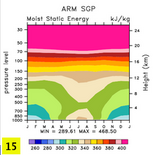b.e20.B1850.f09_g16.pi_control.all.134
and
OBS data (info)

DIAG Set 15 - ARM Sites Annual Cycle Plots

Southern Great Plains (SGP)
T Temperature plot
Q Specific Humidity plot
CLOUD Cloud Fraction plot
MSE Moist Static Energy plot
TMQ Precipitable Water plot
FSDS Downwelling Shortwave Flux at Surface plot
FLDS Downwelling Longwave Flux at Surface plot
TGCLDLWP Total Grid-Box Cloud Liquid Water Path plot
CLDTOT Total Cloud plot
PRECT Precipitation plot
LHFLX Latent Heat Flux plot
SHFLX Sensible Heat Flux plot

T Temperature plot
Q Specific Humidity plot
CLOUD Cloud Fraction plot
MSE Moist Static Energy plot
TMQ Precipitable Water plot
FSDS Downwelling Shortwave Flux at Surface plot
FLDS Downwelling Longwave Flux at Surface plot
TGCLDLWP Total Grid-Box Cloud Liquid Water Path plot
CLDTOT Total Cloud plot

Tropical Western Pacific--Region 1 (TWP1)
T Temperature plot
Q Specific Humidity plot
CLOUD Cloud Fraction plot
MSE Moist Static Energy plot
TMQ Precipitable Water plot
FSDS Downwelling Shortwave Flux at Surface plot
FLDS Downwelling Longwave Flux at Surface plot
TGCLDLWP Total Grid-Box Cloud Liquid Water Path plot
CLDTOT Total Cloud plot

Tropical Western Pacific--Region 2 (TWP2)
T Temperature plot
Q Specific Humidity plot
CLOUD Cloud Fraction plot
MSE Moist Static Energy plot
TMQ Precipitable Water plot
FSDS Downwelling Shortwave Flux at Surface plot
FLDS Downwelling Longwave Flux at Surface plot
TGCLDLWP Total Grid-Box Cloud Liquid Water Path plot
CLDTOT Total Cloud plot

Tropical Western Pacific--Region 3 (TWP3)
T Temperature plot
Q Specific Humidity plot
CLOUD Cloud Fraction plot
MSE Moist Static Energy plot
TMQ Precipitable Water plot
FSDS Downwelling Shortwave Flux at Surface plot
FLDS Downwelling Longwave Flux at Surface plot
TGCLDLWP Total Grid-Box Cloud Liquid Water Path plot
CLDTOT Total Cloud plot

Surface Heat Budget of the Arctic Ocean (SHEBA)
FSDS Downwelling Shortwave Flux at Surface plot
FLDS Downwelling Longwave Flux at Surface plot

Greenland Summit Camp (ICECAPS, 72.3N,38.3W)
U10 10m wind (station data) plot
U10 10m Wind (RACMO) plot
FSDS Downwelling Shortwave Flux at Surface plot
FLDS Downwelling Longwave Flux at Surface plot
FSUS Upwelling Shortwave Flux at Surface plot
FLUS Upwelling Longwave Flux at Surface plot
TMQ Precipitable Water plot
TGCLDLWP Total Grid-Box Cloud Liquid Water Path plot

ERA Interim Greenland Summit (72.3N,38.3W)
LHFLX Surface Latent Heat Flux plot
TS Surface Temperature plot
TMQ Precipitable Water plot
U10 10m Wind plot

DYE2 (Greenland, 66.3N,46.2W)
U10 10m Wind (station data) plot
U10 10m Wind (RACMO data) plot

NGRIP (Greenland, 75.0N,42.2W)
U10 10m Wind (station data) plot
U10 10m Wind (RACMO data) plot

NEEM (Greenland, 77.3N,58.0W)
U10 10m Wind (station data) plot
U10 10m Wind (RACMO data) plot

Humboldt Glacier (Greenland, 78.3N,56.5W)
U10 10m Wind (station data) plot
U10 10m Wind (RACMO data) plot